In the circuit shown in the figure, the current through :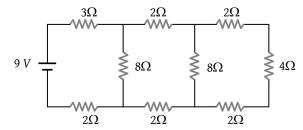1. The 3Ω resistor is 0.50A

2. The 3Ω resistor is 0.25 A

3. The 4Ω resistor is 0.50A

4. The 4Ω resistor is 0.25 A

Concept Questions :-

Combination of resistors
High Yielding Test Series + Question Bank - NEET 2020

Difficulty Level:

There are three resistance coils of equal resistance. The maximum number of resistances you can obtain by connecting them in any manner you choose, being free to use any number of the coils in any way is :

(1) 3

(2) 4

(3) 6

(4) 5

Concept Questions :-

Combination of resistors
High Yielding Test Series + Question Bank - NEET 2020

Difficulty Level:

If in the circuit shown below, the internal resistance of the battery is 1.5 Ω and VP and VQ are the potentials at P and Q respectively, what is the potential difference between the points P and Q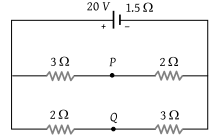(1) Zero

(2) 4 volts (VP > VQ)

(3) 4 volts (VQ > VP)

(4) 2.5 volts (VQ > VP)

Concept Questions :-

Kirchoff's voltage law
High Yielding Test Series + Question Bank - NEET 2020

Difficulty Level:

Two wires of resistance R1 and R2 have temperature coefficient of resistance , respectively. These are joined in series. The effective temperature coefficient of resistance is :

(1) $\frac{{\alpha }_{1}+{\alpha }_{2}}{2}$

(2) $\sqrt{{\alpha }_{1}{\alpha }_{2}}$

(3) $\frac{{\alpha }_{1}{R}_{1}+{\alpha }_{2}{R}_{2}}{{R}_{1}+{R}_{2}}$

(4) $\frac{\sqrt{{R}_{1}{R}_{2}{\alpha }_{1}{\alpha }_{2}}}{\sqrt{{R}_{1}^{2}+{R}_{2}^{2}}}$

Concept Questions :-

Combination of resistors
High Yielding Test Series + Question Bank - NEET 2020

Difficulty Level:

Two cells of equal e.m.f. and of internal resistances, r1, and ${r}_{2}\left({r}_{1}>{r}_{2}\right)$ are connected in series. On connecting this combination to an external resistance R, it is observed that the potential difference across the first cell becomes zero. The value of R will be :

(1) ${r}_{1}+{r}_{2}$

(2) ${r}_{1}-{r}_{2}$

(3) $\frac{{r}_{1}+{r}_{2}}{2}$

(4) $\frac{{r}_{1}-{r}_{2}}{2}$

Concept Questions :-

Grouping of cells
High Yielding Test Series + Question Bank - NEET 2020

Difficulty Level:

When connected across the terminals of a cell, a voltmeter measures 5V and a connected ammeter measures 10 A of current. A resistance of 2 ohms is connected across the terminals of the cell. The current flowing through this resistance will be :

(1) 2.5 A

(2) 2.0 A

(3) 5.0 A

(4) 7.5 A

Concept Questions :-

Kirchoff's voltage law
High Yielding Test Series + Question Bank - NEET 2020

Difficulty Level:

In the circuit shown here, E1 = E2 = E3 = 2 V and R1 = R2 = 4 ohms. The current flowing between points A and B through battery E2 is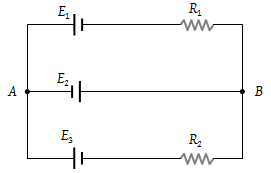(1) Zero

(2) 2 amp from A to B

(3) 2 amp from B to A

(4) None of the above

Concept Questions :-

Grouping of cells
High Yielding Test Series + Question Bank - NEET 2020

Difficulty Level:

In the circuit shown below E1 = 4.0 V, R1= 2 Ω, E2 = 6.0 V, R2 = 4 Ω and R3 = 2 Ω. The current I1 is :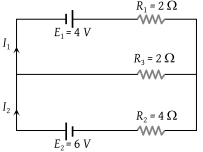(1) 1.6 A

(2) 1.8 A

(3) 1.25 A

(4) 1.0 A

Concept Questions :-

Grouping of cells
High Yielding Test Series + Question Bank - NEET 2020

Difficulty Level:

The potential difference across 8 ohms resistance is 48 volts as shown in the figure. The value of potential difference across X and Y points will be :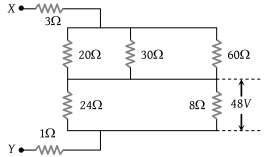(1) 160 volt

(2) 128 volt

(3) 80 volt

(4) 62 volt

Concept Questions :-

Combination of resistors
High Yielding Test Series + Question Bank - NEET 2020

Difficulty Level:

Two resistances R1 and R2 are made of different materials. The temperature coefficient of the material of R1 is α and of the material of R2 is –β. The resistance of the series combination of R1 and R2 will not change with temperature, if R1/ R2 equals :

(1) $\frac{\alpha }{\beta }$

(2) $\frac{\alpha +\beta }{\alpha -\beta }$

(3) $\frac{{\alpha }^{2}+{\beta }^{2}}{\alpha \beta }$

(4) $\frac{\beta }{\alpha }$

Concept Questions :-

Derivation of Ohm's law